# Orthogonal basis

(diff) ← Older revision | Latest revision (diff) | Newer revision → (diff)

A system of pairwise orthogonal non-zero elementsof a Hilbert space, such that any elementcan be (uniquely) represented in the form of a norm-convergent seriescalled the Fourier series of the elementwith respect to the system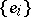. The basisis usually chosen such that, and is then called an orthonormal basis. In this case, the numbers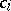, called the Fourier coefficients of the elementrelative to the orthonormal basis, take the form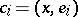. A necessary and sufficient condition for an orthonormal systemto be a basis is the Parseval–Steklov equality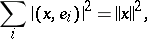for any. A Hilbert space which has an orthonormal basis is separable and, conversely, in any separable Hilbert space an orthonormal basis exists. If an arbitrary system of numbers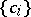is given such that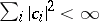, then in the case of a Hilbert space with a basis, the seriesconverges in norm to an element. An isomorphism between any separable Hilbert space and the space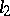is established in this way (Riesz–Fischer theorem).

How to Cite This Entry:
Orthogonal basis. Encyclopedia of Mathematics. URL: http://encyclopediaofmath.org/index.php?title=Orthogonal_basis&oldid=14572
This article was adapted from an original article by V.I. Sobolev (originator), which appeared in Encyclopedia of Mathematics - ISBN 1402006098. See original article